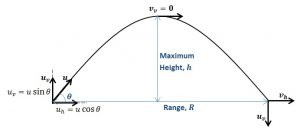# What is Projectile Motion? – Types, Derivation, Formulae 2023

Before we delve into the topic, let us first find out what a Projectile is. It is an object that has been thrown into space, on which gravity acts as an opposing force. Moreover, you should also remember that there are other forces acting on the projectile, but they are very minimal. And the acting of different forces as well as the magnitude of gravitational forces depends on the path taken by the Projectile, which is defined as a trajectory. For instance, when a ball is thrown into the air, it will follow a specified trajectory and will fall down after a certain point. Because of the gravitational forces, this ball can be considered a projectile.

## What is a Projectile Motion?

Projectile Motion is defined as a path taken by a particle or object that is thrown indirectly toward the earth’s atmosphere. Then the object moves in a curved path, and it faces constant acceleration toward the center of the earth, due to gravitational forces. And the path taken by the object is stated as a projectile, and the motion of an object is called Projectile Motion.

## Types of Projectile Motion

Moreover, there are three different types of projectile motions, such as Oblique Projectile Motion, Horizontal Projectile Motion, and Projectile Motion on an Inclined Plane.

##### Oblique Projectile Motion

When the motion of an object, is Thrown into the air from the ground, the said object is pointed vertically downwards due to the gravitational force acting on it. Then this type of motion is Oblique Projectile Motion.

##### Horizontal Projectile Motion

As the title itself suggests, the projectile motion which acts on a horizontal plane is defined as the Horizontal Projectile Motion.

##### Projectile Motion in Inclined Plane

Similar to the Horizontal projectile motion, the motion of a projectile acting on an inclined plane is stated as the Projectile Motion in an Inclined Plane.

## Parabolic Motion of ProjectileFrom the above diagram, you can observe that regardless of the angle or inclinational of the projectile, it always results in a parabolic motion. This is mainly because of the gravitational force that acts on it.

Moreover, in order to calculate different types of parameters, we need to use various types of differential equations of motion or different types of projectile motion equations.

$$v=u-gt$$

$$s=ut- \frac{g t^{2} }{2}$$

$$v^{2} = u^{2} -2gs$$

In which, u = Initial Velocity

g = acceleration due to gravity

v = Final Velocity

s = Displacement

t = Time

## Conclusion

The above article will help you get a complete understanding of Projectile Motion, the equation of trajectory in projectile motion, different types of projectile motions, etc. Now if you have more doubts or you are struggling with any other concepts or chapters in Physics. Then the online interactive classes offered by Tutoroot Platform might help you out. Mainly because with these classes, the students will have expert staff guidance, access to some of the best test books, educational guides, and many more.